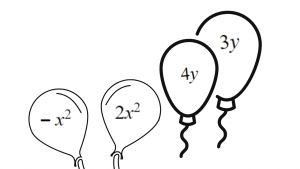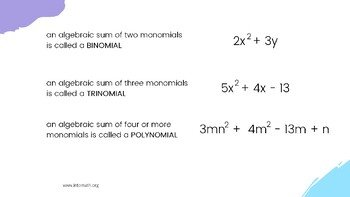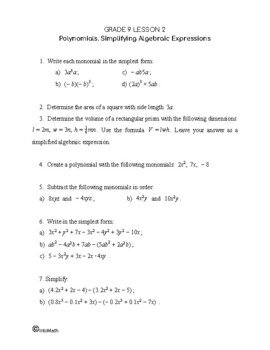Video LessonCA$2.00 In this lesson you will learn about different types of algebraic expressions: monomials, binomials, trinomials and polynomials. You will see how two monomials could be multiplied or added/subtracted. For example 2m × 3m = 6m² or 4m + 12m = 16m. In math, a polynomial is an expression consisting of coefficients and variables. These coefficients and variables are called “terms”. Terms in a polynomial are separated by operations of addition, subtraction, multiplication. Variables have only positive integer exponents.Grade 9 Workbook (14 pages with answers) CA$4.00

This lesson also explains how algebraic expressions with brackets can be simplified by distributing a monomial in front of the bracket by every term inside the bracket. For example, an expression 3(2s -3) could be expanded to 6s – 9.

This may be followed by collecting like terms (monomials within polynomials that only differ from each other by their coefficient) if there are more than one bracket.